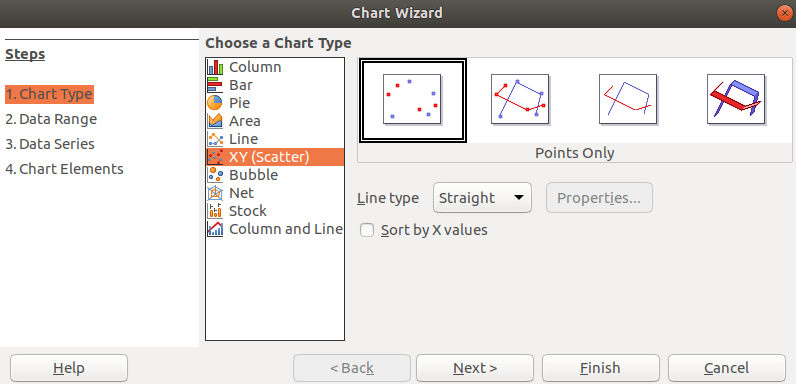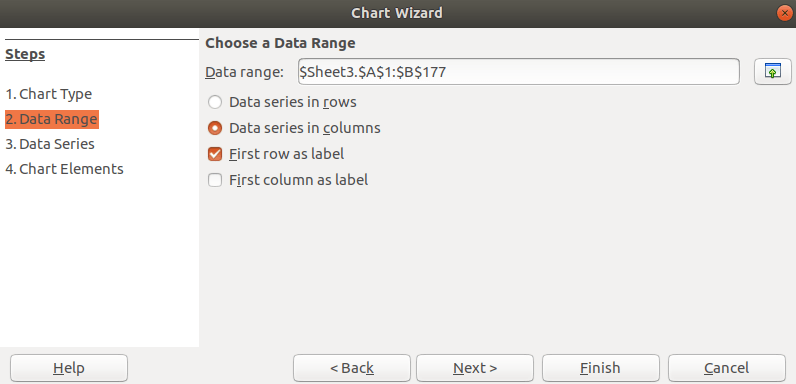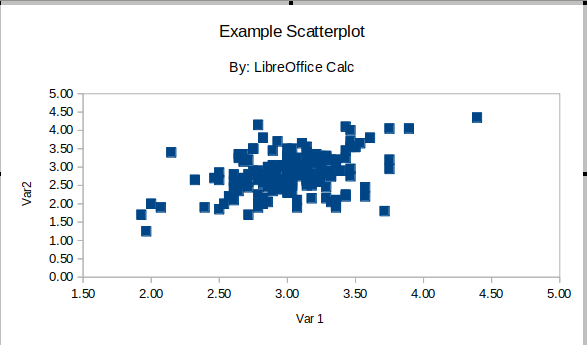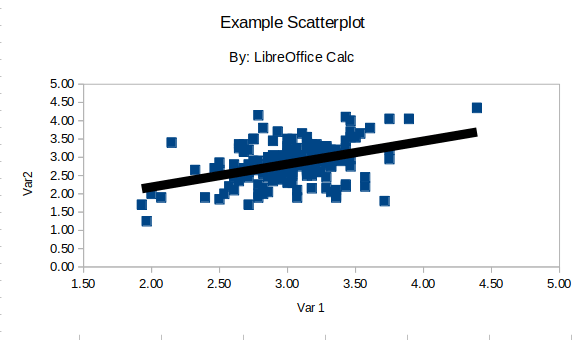# Scatterplot in LibreOffice CalcA scatterplot is used to observe the relationship between two continuous variables. This post will explain how to make a scatterplot and calculate correlation in LibreOffice Calc.

ScatterplotIn order to make a scatterplot you need to columns of data. Below are the first few rows of the data in this example.

 Var 1 Var 2 3.07 2.95 2.07 1.90 3.32 2.75 2.93 2.40 2.82 2.00 3.36 3.10 2.86 2.85

Given the nature of this dataset, there was no need to make any preparation.

To make the plot you need to select the two column with data in them and click on insert -> chart and you will see the following.Be sure to select the XY (Scatter) option and click next. You will then see the followingBe sure to select “data series in columns” and “first row as label.” Then click next and you will see the following.There is nothing to modify in this window. If you wanted you could add more data to the plot as well as label data but neither of these options apply to us. Therefore, click next.In this last window, you can see that we gave the chart a title and label the X and Y axes. We also removed the “display legend” feature by unchecking it. A legend is normally not needed when making a scatterplot. Once you add this information click “finish” and you will see the following.There are many other ways to modify the scatterplot, but we will now look at how to add a trend line.

To add a trend line you need to click on the data inside the plot so that it turns green as shown below.Next, click on insert -> trend line and you will see the followingFor our purposes, want to select the “linear” option. Generally, the line is hard to see if you immediately click “ok”. Instead, we will click on the “Line” tab and adjust as shown below.All we did was simply change the color of the line to black and increase the width to 0.10. When this is done, click “ok” and you will see the following.The  scatterplot is now complete. We will now look at how to calculate the correlation between the two variables.

Correlation

The correlation is essentially a number that captures what you see in a scatterplot. To calculate the correlation, do the following.

1. Select the two columns of data
2. Click on data -> statistics -> correlation and you will see the following3. In the results to section just find a place on the spreadsheet to show the results. Click ok and you will see the following.

 Correlations Column 1 Column 2 Column 1 1 Column 2 0.413450002676874 1

You have to rename the columns with the appropriate variables. Despite this problem the correlation has been calculated.

Conclusion

This post provided an explanation of calculating correlations and creating scatterplots in LibreOffice Calc. Data visualization is a critical aspect of communicating effectively and such tools as Calc can be used to support this endeavor.